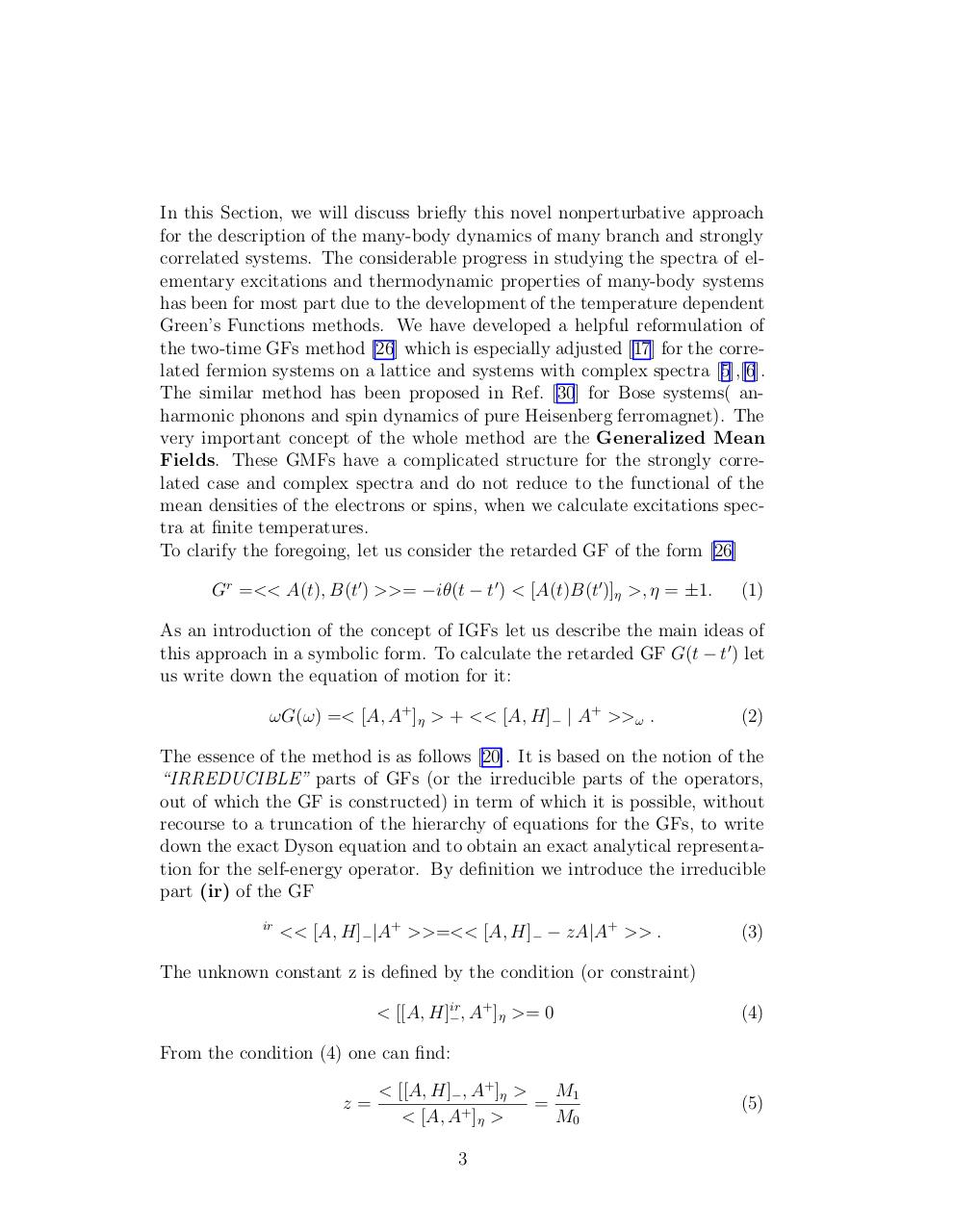# Quantum Magnetism and Spin Fermion Models.pdfPage 1 2 3 45637

#### Text preview

In this Section, we will discuss briefly this novel nonperturbative approach
for the description of the many-body dynamics of many branch and strongly
correlated systems. The considerable progress in studying the spectra of elementary excitations and thermodynamic properties of many-body systems
has been for most part due to the development of the temperature dependent
Green’s Functions methods. We have developed a helpful reformulation of
the two-time GFs method  which is especially adjusted  for the correlated fermion systems on a lattice and systems with complex spectra ,.
The similar method has been proposed in Ref.  for Bose systems( anharmonic phonons and spin dynamics of pure Heisenberg ferromagnet). The
very important concept of the whole method are the Generalized Mean
Fields. These GMFs have a complicated structure for the strongly correlated case and complex spectra and do not reduce to the functional of the
mean densities of the electrons or spins, when we calculate excitations spectra at finite temperatures.
To clarify the foregoing, let us consider the retarded GF of the form 
Gr =&lt;&lt; A(t), B(t0) &gt;&gt;= −iθ(t − t0) &lt; [A(t)B(t0)]η &gt;, η = ±1.

(1)

As an introduction of the concept of IGFs let us describe the main ideas of
this approach in a symbolic form. To calculate the retarded GF G(t − t0) let
us write down the equation of motion for it:
ωG(ω) =&lt; [A, A+]η &gt; + &lt;&lt; [A, H]− | A+ &gt;&gt;ω .

(2)

The essence of the method is as follows . It is based on the notion of the
“IRREDUCIBLE” parts of GFs (or the irreducible parts of the operators,
out of which the GF is constructed) in term of which it is possible, without
recourse to a truncation of the hierarchy of equations for the GFs, to write
down the exact Dyson equation and to obtain an exact analytical representation for the self-energy operator. By definition we introduce the irreducible
part (ir) of the GF
ir

&lt;&lt; [A, H]−|A+ &gt;&gt;=&lt;&lt; [A, H]− − zA|A+ &gt;&gt; .

(3)

The unknown constant z is defined by the condition (or constraint)
+
&lt; [[A, H]ir
− , A ]η &gt;= 0

(4)

From the condition (4) one can find:
z=

M1
&lt; [[A, H]−, A+ ]η &gt;
=
+
&lt; [A, A ]η &gt;
M0
3

(5)